Gravity

By Leonard Van Zanten May 10 2019

Note" (This essay was send to the gravity research foundation in competition. It should have gotten first price since it is the only essay of any truth, or even to make any sense.  All others are fantasy, but all five were awarded to various universities for their trash - where if one seeks an education he will be brainwashed to fantasies

Then they had the nerve to ask me the year after, if I wanted to join the competition again, when their awards are rigged for the most incompetent. These people are not interested in the truth, nor what gravity really is, their only aim is to relish in gross stupidity. And for that their hides will burn in the everlasting fire.

Abstract

This essay introduces a realistic look at our laws of gravity, and the effects thereof, and it goes beyond to reveal how and by what gravity comes about in the fundamentals of nature upon which the earth and all spheres rest.

Keywords

Gravity; Motion; Inertia.

Essay

1. Written for the Gravity Research Foundation 2019 awards for Essays on Gravitation.  Submitted March 5 2019

2. Our weight upon the scale is said to be the force of gravity, and right we are, but our rotation places an additional force of gravity upon us. For the example: By the law of Newton, (Ref 1)

3. Radius is: 4000 miles X 5280-ft = 21,120,000-ft. Velocity near the equator: 1000-mph x 5280 = 5,280.000-ft/hr : 60 = 88,000-ft/min : 60 = 1,467-ft/sec.

4. Accordingly 165 X 1457 = 242,055 X 1467 = 355.094,685 : 21,120,000 = 16.81-lb.

5. The centrifugal inertia upon that person traveling at 1000-mph is thus 16.81 pounds, and every ounce of that is augmented by the gravitational force of the earth. That amount of gravity then cannot possibly show up on the scale since it are two impacts off-setting one another.

6. But the conclusion here is that the full amount of gravitational force upon a person at 165-lb is 181.81/lb of g.

7. Then as to “why” we have to square the force of velocity, lies in the combination of inertia, the 165/lb of his angular inertia as weight upon the scale plus the added inertia of our movement in the linear.

8. As one moves, his velocity in the linear compounds with his angular momentum, the grand total of the atoms in their movement. As therefore the linear multiplies it does so upon the angular coming to a four-fold force.

9. Gravity now as a force has a limited range, and to prove this; the data on the Hubble space telescope as given:  Gross weight 24.500 lb.  Orbital altitude 353 miles.  Orbital velocity 17.500 mph.

10. And to do this in whole numbers:  24.500 x 25.666 = 628,817.000 x 25,666 = 16,139,217,122.000 divided by 22,983,840 = 702,198.46 lb, divided by 24.500 = 28.66.

11. This makes the Hubble telescope to have a g/force on it 28.66 times as much as it read upon the scale upon earth, since 702.198.46 divided by its scale weight comes to 28.66.  The factor of gravity 353 miles above the earth therefore is 28.66 and its acceleration if it were to fall would be 28.66 ft/sec/sec. (Ref 3)

12.  When therefore we deduct the 28.66 from 32.174 (at sea level) it comes to a decrease of 3.514 in those 353 miles, and this divided by the 353 comes to 0.009.954.674 per mile.

13. Then by dividing the sea level factor of 32.174 by the decrease per mile, comes to 3.232 miles, at which point the factor of gravity is zero, and the acceleration would be zero ft/sec/sec.

14. With our moon at a distance of some 240.000 miles, how can it possibly be held gravitational?

15. Gravity thus is but a local force, and at that the female of force to her masculine man named magnetic, Even as it is by the power of magnetic that we are drawn to the earth, with gravity providing the intricate precession whereby all substances may be embraced by those masculine arms of her man to draw it down to its center at a fixed precessional rate.

16. Next we must come to understand the mechanics of nature, in particular the nature and effect of inertia, from where we come to how and why the earth’s magnetic force picks up on it.  Evidence that all atoms are in rotational motion lends itself to the fact that all mass displays inertia, and inertia comes about by movement..

17. The atoms as angular momentums thus present the angular inertia, and once put into linear motion will present the linear inertia. Therefore when we take the law of gravity to put an object into orbit and we wish to discover its resistance in the centrifugal as well as the gravitational hold upon it, we must square the force.

18. And while we have the acceleration of gravity at 32.174 ft/sec/sec (sea level) (Ref 1) it is factually a factor of inertia.

19. Now we must adapt a few terms to ourselves, the ever rotation of the atoms shall be the “A” factor with the linear movement as the “L” factor. The twofold factor of inertia therefore is A, and L. Then recall how the pull of gravity is our weight plus the additional g of our circular movement.

20. The type of gravity affecting our weight then is different from the gravity that keeps us in a uniform circular movement. The gravity for weight is vectored to the center of the earth, while the additional g is vectored to the axis of the earth.

21. The first is born forth because the earth is a magnet, meaning an endless array of figure eights of force that by their particular nature or coordinate quells all things to its center, a force in eight at full equilibrium.

22. But when we take on a circular movement at a location in Canada our vector is no longer to the center of the earth but upon the axis of the earth directly beneath our feet where the norm of gravity augments our centrifugal impact by a factor of speed to radius.

23. And so we come to our atomic gyros and what role they play in the phenomena known as gravity. A gyro seems to defy gravity, in reality a gyro effects inertia, to stay put, to stay in place, but when acted upon by any linear movement, it becomes known as a torque upon it, and they begin to spiral..

24. Then we come to the ever-fundamental movement in nature, like between two magnets. These so-called lines are susceptible to all that is construed as a medium, evidence to this is found with all waves in reflection and refraction.

25. But how are these lines of magnetic movement that pass along all atoms able to take a hold of them so as to draw them to their center of equilibrium, to exhibit gravity?  The secret lies in entwining, for one entity or movement to entwine itself around the other, much like the woman embracing her man who then will take her home.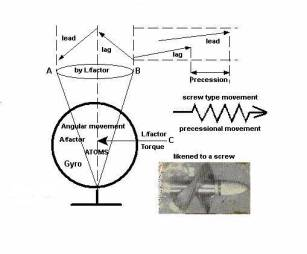Figure 1.  Gravity as an inclination to movement becomes entwined with the magnetic potential pulling it to its center of the force.

1. And to make it simple; by Figure 1, when the ever angular “C” (atomic gyros) are displaced, that is - set into linear motion, they tends to precess, noted A-B. It then is by this precession whereby it comes entangled with the lines of movement, embracing them whereby of course the substance is drawn down. (quell)

2. Formerly with the atomic gyro at a standstill the lines merely pass, but once set in motion it is like a matrimony. This therefore is important to remember that a gravitational movement does not proceed unless there is movement into the linear after which it essentially screws itself down by virtue of the magnetic lines (fabric) of movement.

3. It is for that reason that I drew a lead/lag scenario by which anything into an angular precession also moves into the linear, the screw of a propeller giving a likeness thereto.

4. If we ever wondered why all objects of varied weights fall with the same rate of acceleration, here is the answer; a nut will go down along a treated bolt by the angular moment of the tread, irrelevant as to how large that nut may be.

5. The speed of acceleration then is by the angular moment of the g/factor.  The greater the g/factor, the greater the acceleration will be.

6. And to go over this once again by Figure 2, at K assume any number of atoms that for their “inclination” towards precession trace out the pattern of a circle noted at P.  These by their number then produce an "overall" pattern angularly induced. The oval at P represents precession, P2 is a top view with P3 a side view.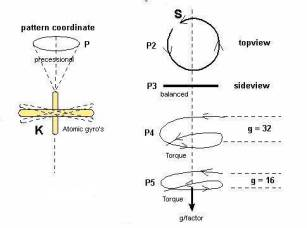Figure 2. Pattern of precession whereby objects are moved according to the torque upon them expressed as “The factors of gravity” towards acceleration.

1. As thus a torque is placed upon the mass at K, the coordinate movement changes in this manner, at P2 - the leading end S, dips under (Precesses) so that now instead of a flat circle it takes on the resemblance of a screw as shown by P4, and P5.

2. The factor of gravity may then be illustrated here in the torque by the degree thereof. At P4, the strength of the force being greatest shows itself by the figure of 32, which is in lb.

3. While at much higher altitudes, the g/factor having been reduced to 16 (in lb) the torque is seen to be equally less.  This then is accurate, for if from this, one construes that at a g/factor of 32 the rate of acceleration is 32 ft/sec/sec, while at 16 the rate of acceleration is at 16 ft/sec/sec, he is quite correct.

4. There is of-course a lot more to this but so far it does represent the “principle fashion” how all substances come into the embrace of the 3M, the ever-magnetic movement that is everywhere.

5. But none of it would become gravitational unless it is first set in motion, any linear motion, and since all things are always moving so gravity is found near any celestial body.

6. Consequently the power of gravity is magnetic, with gravity as such the “inclination” towards downward movement in and by the inclination to precession forming an overall pattern, or coordinate that is like a nut on a bolt.

7. And so now – in principle - we know the secret of gravity and are ready for the next step to behold the greater formations.

Gravity beyond earth

1. By illustration Figure 3, we will first assume our earth at a “stand still”, and to place a force upon it at point H, to drive it away in a straight on trajectory towards M to the tune of 15 km/sec.

2. Then in going back to basics - all that angular movement of the fundamental parts of any mass have this tendency, to resist being moved, and once in motion to resist being stopped, as well to resist any change in direction.

3. Thus with the earth at an idle the angular momentum of its mass may be likened to a gyro at balance.  Here the A/factor is active, but the L/factor is at zero, wherefore the atomic gyros are at what we call in a state of balance.

4. Then as we began to move the earth to accelerate it - the L/factor stepped in, placing a torque upon that angular motion, and reaching our maximum velocity of 15 km/sec, the force at figure 3-H, is abandoned, while the earth continues to move at its now constant velocity in a straight trajectory, direction “M”.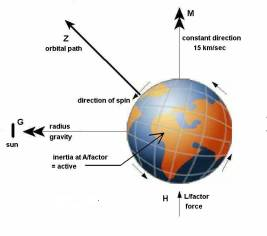Figure 3. The earth susceptible to gravity by movement like unto a gyro upon which a torque is placed that as such works itself into the magnetic fabric.

1. At this point there is not as yet a vector, nor therefore an orbit.   As thus we visualize that earth now without any power acting on it happily moving at its said velocity, the only real action occurring within at those fundamental levels is the A/factor, the atomic angular movement that can never be removed.

2. Yet now for its added  linear movement it has a degree of overall precession upon its general movement. This part though it is not as yet an empowered inclination towards gravity, it is nonetheless a factor upon which the 3M, the magnetic is susceptible.

3. The earth at this point has no inclination to slow down, for even as much as it resisted the acceleration, it equally resists any deceleration.

4. And while thus far there is not any magnetic force to be found, the earth continues on its straight trajectory oblivious to anything, nor therefore any gravity. It is no more than a mass moving in space.

5. Then let us have that circular movement, which when twisted by 180 degrees over itself becomes what we call - "magnetic."   And here I must provide evidence that the format of magnetic is not two circles but a single circle in the design of a figure eight

6. By Figure 4 when steel filings are placed around one magnet the pattern of the magnetic lines are circular (solid lines) but when a second magnet (magnet B) is quickly drawn away from it as shown the circular lines become elongated.

7. The evidence to the eight of force is in this that not only the lines between the two magnets become elongated but those on the opposite side of magnet “A” as well in equal proportion.

8. The same thing happens to our “tides” as the moon pulls on one side there are always two equal tides on both sides of the earth, because magnetic is a singular format by the pattern of an eight.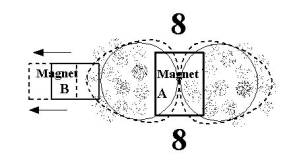Figure 4. Evidence of the magnetic format to exist by a figure of eight

1. In order now to secure a magnetic entity one needs a body to implement it upon, which as such then becomes a vector.  And for our first consideration, let it be the sun, at point G, in Figure 3. The 3M of that sun then looks at our A/factor and observes a precession of it, and for its particular nature of movement being susceptible to that precession it embraces it.

2. If then we think, it has a good hold of us, we are partly in error, for as long as the earth does not attempt to distance itself from the sun, it is simply an embracing.

3. But the instant the earth does move away from the sun, then the torque kicks in, On the one hand the magnetic tentacles tighten in on those angular coordinates, while the earth for its mass and speed place a heavy load upon them. And so here comes the equilibrium when both forces come equal to one another.

4. Now that we have our earth bound to the sun at a yearly clip around it, pulling it inwards to itself with the earth resisting that pull in its velocity constantly attempting to take a straight course, so it takes on an orbit. The next essential factor then is to create a magnetic entity upon the earth so that the earth may take on the shape of a sphere.

5. For in placing a magnetic entity on it, it likewise, like the sun at first, - is susceptible to the very same angular precessional movement, and as such holds it in place.

6. But here, there is a distinct difference in that the former magnetic vector was 93 million miles away with only its tentacles holding us that now is seated right upon that very mass itself. That then centers itself upon that mass by virtue of its own design, remembering how that coordinate is in all respects a series of figures of eight

7. Accordingly there is now a force of gravity upon the earth that can be read upon the scale. Now then we are going to give the earth a period of rotation, but in doing so - the substance of the earth set into linear movement around an axis is also wishing to maintain a straight course being vectored to earth’s axis.

8. Note clearly however that in this case the vector is not the center of the earth, but its axis, the point to which all of that centrifugal mass is drawn. That is a vector some 8000 miles long, towards which the balance of our gross weight in g/force is drawn.

9. That same scenario then occurs here that previously occurred when we placed a rope from the sun upon the earth to force it into an orbital path, - the 3M placing a torque - relevant to the axis of the earth.

10. All rotating bodies have an axis, magnetism alone presents a center, something that for its coordinate does what nothing else in nature does to present a single point, be it stationary or rotating, as it must since its coordinate is that of an eight.

11. And as such, by its design, or coordinate as I call it, it produces a linear power, and a pherical design.  Think about this how unique that magnetic entity is, to perform what nothing else in nature can perform, and how for its unique ability all things in nature are formed by it, as well as sustained by it.

12. And here again I must provide evidence in how linear movement is born forth. By Figure 5  at “A” there is only angular momentum, but when that angular is twisted half over becoming a movement in eight there is an outgoing movement at the north with an ingoing movement at the south.

13. The circular as it comes around at “X” continues on to “Y”, it as such provides for linear movement at its polar areas.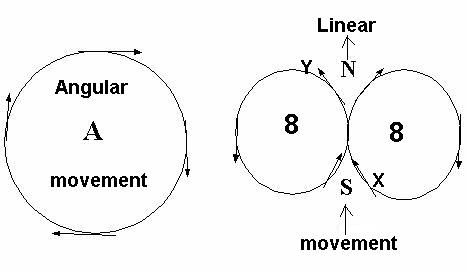Figure 5.  Linear movement born forth by the figure eight of magnetic.

1. The angular inertia of the earth thus has to do with a torque in two different aspects, one of which is vectored to the sun, while the second is vectored to the axis of the earth.

2. And as such being vectored differently, they not only act, as were they independent of one another, but factually are independent of one another.

3. And for a third aspect is that basic angular inertia of the 3M, the magnetic entity that inhibits earth, which as such is vectored to the very center of the center of the earth.

4. As thus we came to question, how weight remains the same all around the earth, and yet by the centrifugal impact - strongest at its equator, there is an additional gravitational pull, the answer is.

5. Because the impact of earth’s rotation does not as such register itself upon our scales, but is nonetheless present in and by the centrifugal impact of our daily rotation.

6. The real answer here lies in the vectors. The sun has its own, -- our rotation has its own, -- and our mass has its own. Three different vectors upon one and the same angular inertia.  Therefore at any given latitude the factor of gravity remains constant, changing only for a change in altitude, a change that brings us, not necessarily further from the axis, but from the very center of the center of the earth, the vector to which it applies.

7. How therefore will the gravitational pull that is vectored to earth's axis show up on our scales, when it is altogether vectored differently?  Yet it is very much present, only in order to embark upon it, we must calculate the same.

8. The magnetic fabric that inhibits the earth does not have anything to do with centrifugal wherefore its pull can be read upon a scale, while anything in a uniform circular motion effects that which we call centrifugal, that then at all times is subject to and augmented by gravitational implications, and as such cannot show up on a scale.

9. Newton’s law therefore by which the g/force in the centrifugal are calculated does not apply to our weight, nor therefore to the acceleration of g.

10. The 32 ft/sec/sec has no relevance to the law of Newton, nor therefore to the center of the earth, other than its axis since that law only applies to anything under the duress of what is called centrifugal.

11. While our weight as a g/force is not as such by centrifugal, if it were there would be no weight to read upon any scale, all because gravity augments all centrifugal inertia. When an upwards force is equally offset by a downward force the net result to be read is zero.

12. There now are ways to double check my teaching, at a midway point between a pole and the equator with our scale weight we are drawn directly to the center of the earth, while the additional g upon us is in line to the axis the earth. Our upright position thus is always to two vectors.

13. There is thus this experiment one could perform, how our upright position on the earth is tilted by that effect, and also by the effect of the moon upon us, for as the magnetic lines between them become elongated raising the waters to a tide, we ourselves are drawn with that elongation.

14. And this can be done at either or both sides of the earth, under and away from the moon, since all magnetic fabric is by a coordinate resembling the figure of eight. Here thus is the means to test my statements, since I have not been promoting a theory, but facts as the Almighty Lord taught me. And you will find all these things to be quite correct.

Conclusion

1. It simply is not possible for any man to discover the nature of gravity that as such pronounces the foundations of the earth, if anyone was so able he would be the death of all mankind for thus spoke the Creator of the earth: (Jer 31:37)

2. "If the heavens above can be measured, and the foundations of the earth below can be explored, then I will cast off all the descendants of Israel for all that they have done, says the Lord."

3. Then again it is written by Esdras 6:10: “Therefore when it speaks, be not afraid, for the word is of the end and the foundations of the earth are understood.  For at the sound of these things they will tremble and be moved, for they know that the end entails change."

4. I now did not discover the foundations of the earth, nor gravity, nor any of nature’s fundamentals, all of them were taught unto me.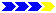Next page

References

Ref-1 https://www.vcalc.com/wiki/vCalc/g+%28acceleration+due+to+gravity+at+sea+level+-+SI+units%29

http://www.physicsclassroom.com/Class/newtlaws/U2L3a.cfm